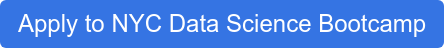# Introducing C/C++ for Statistical Computing and Integration with R (1/3)

## Introducing C/C++ for Statistical Computing and Integration with R (1/3)

Updated: Jul 16, 2014

Many thanks go to SumAll for providing the space for this event!

Special thanks go to Paul Trowbridge for giving such a great workshop!

---------------------------------------------------------------------------------------------

NYC Data Science Academy is offering an relative courses:

---------------------------------------------------------------------------------------------

Video:

Click here to subscribe to our channel! NYC Data Science Academy!

---------------------------------------------------------------------------------------------

## Meetup Announcement:

### Speaker: Paul Trowbridge.

Paul Trowbridge is a graduate student in statistics at Rutgers University and an adjunct faculty member with the Center for Advanced Digital Applications at NYU. He teaches statistical computing with C++ with the New York City Data Science Academy and co-organizes the New York Data Visualization meetup. He was worked on projects in the epidemiology of sexually transmitted infections, microsimulation modeling of urban behavior, fMRI research, and international dispute resolution.

### Outline:

This presentation and workshop introduced statistical computing with C/C++ and integrating compiled C++ code with R.  This is the first workshop in a series of three culminating in coding advanced statistical methods in C++.

In this first workshop, Paul introduced statistical programming with C/C++, the .C, .Call and Rcppinterfaces between C/C++ and R.  Through a series of introductory examples, Paul introduced C++ programming for statistical computing. Exercises also were provided for the participants to work on illustrating the concepts and methods introduced in the workshop.

The goal of this workshop is to introduce enough of the basics of C++programming for statistical computing and interfacing compiled code with R so that workshop participants will be able to code simple statistical analyses in C++ and integrate their code with R.

### Preparation:

- A C++ compiler (g++ works great and is free)
- R
- A text editor

---------------------------------------------------------------------------------------------

### Materials:

#### Source code:

- C++:

1. angle.cpp

```# include <R.h>
# include <Rinternals.h>
# include <Rmath.h>
using namespace std;
extern "C" {
void anglevec(int* size, double* x, double* y, double* result) {

// compute dot product
double dxy = 0.0;
for(int i = 0; i < *size; i++){
dxy += x[i] * y[i];
}

// compute vector lengths
double dxx = 0.0;
double lx = 0.0;
double dyy = 0.0;
double ly = 0.0;

for(int i=0; i < *size; i++){
dxx += x[i] * x[i];
}
lx = sqrt(dxx);

for(int i=0; i < *size; i++){
dyy += y[i] * y[i];
}
ly = sqrt(dyy);

// compute angle between vectors
*result = acos(dxy / (lx * ly));
}
}```

2. dotProd.cpp

```# include <R.h>
# include <Rinternals.h>
# include <Rmath.h>

extern "C" {
void dotProd(int* size, double* x, double* y, double* result) {
for(int i = 0; i < *size; i++){
*result += x[i] * y[i];
}
}
}```

3. hello.c

```#include <R.h>

void hello(int* n)
{
int i;
for(i=0; i < *n; i++) {
Rprintf("Hello, world!n");
}
}```

4. nr.cpp

```# include <R.h>
# include <Rinternals.h>
# include <Rmath.h>

using namespace std;

extern "C" {
void nr(double* x0, double* tol, int* maxit, int* niter, double* root) {
int iter = 0;
double diff = 1.0;
while ( (diff > *tol) && (iter <= *maxit) ) {
*root = *x0 - ( (pow(*x0,2)-612) / (2**x0) );
diff = fabs(*root - *x0);
*x0 = *root;
iter++;
}
*niter = iter;
}}```

5. pearson.cpp

```# include <R.h>
# include <Rinternals.h>
# include <Rmath.h>
# include <math.h>

using namespace std;

extern "C" {
void pearson(int* size, double* x, double* y, double* result) {
double mx, my, xt, yt;
double xx=0.0, yy=0.0, sxx=0.0, syy=0.0, sxy=0.0;
// compute the means
for(int i=0; i < *size; i++){
xx += x[i];
yy += y[i];
}
mx = xx / *size;
my = yy / *size;

for(int i=0; i < *size; i++){
xt = x[i] - mx;
yt = y[i] - my;
sxx += xt*xt;
syy += yt*yt;
sxy += xt*yt;
}
*result = sxy / (sqrt(sxx*syy));
}
}```

6. pearson2.cpp

```# include <R.h>
# include <Rinternals.h>
# include <Rmath.h>

using namespace std;

extern "C" {
void pearson2(int* size, double* x, double* y, double* value, double* tstat, double* pval) {
double mx, my, xt, yt;
double xx=0.0, yy=0.0, sxx=0.0, syy=0.0, sxy=0.0;
// compute the means
for(int i=0; i < *size; i++){
xx += x[i];
yy += y[i];
}
mx = xx / *size;
my = yy / *size;

for(int i=0; i < *size; i++){
xt = x[i] - mx;
yt = y[i] - my;
sxx += xt*xt;
syy += yt*yt;
sxy += xt*yt;
}
*value = sxy / (sqrt(sxx*syy));
*tstat = *value*(sqrt((*size-2)/(1-pow(*value,2.0))));
*pval = 2*pt(*tstat,*size-2,1,0);
}
}```

- R:

1. angle.r

```angle2 <- function(x,y){
size <- length(x)
result <- 0.0
cfun <- .C("anglevec", size=as.integer(size),x=as.double(x),y=as.double(y),result=as.double(result))
return(cfun[["result"]])
}```

2.dotProd.r

```dyn.load("dotProd.so")
dotprod <- function(x,y){
# get length of x,y
size <- length(x)
result <- 0.0
.C("dotProd", as.integer(size),as.double(x),as.double(y), as.double(result))
}
dotprod2 <- function(x,y){
size <- length(x)
result <- 0.0
cfun <- .C("dotProd", size=as.integer(size),x=as.double(x),y=as.double(y),result=as.double(result))
return(cfun)
}
dotprod3 <- function(x,y){
size <- length(x)
result <- 0.0
cfun <- .C("dotProd", size=as.integer(size),x=as.double(x),y=as.double(y),result=as.double(result))
return(cfun[["result"]])
}```

3. hello2.r

```hello2 <- function(n) {
.C("hello", as.integer(n))
}```

4. nr2.r

```nr2 <- function(x0, tol=1e-5, maxit=30){
cfun <- .C("nr",x0=as.double(x0), tol=as.double(tol), maxit=as.integer(maxit), niter=integer(1), root=double(1))
return(list=c(root=cfun[["root"]],niters=cfun[["niter"]]))
}```

5. pearson2.r

```pearson2 <- function(x,y){
size <- length(x)
cfun <- .C("pearson", size=as.integer(size),x=as.double(x),y=as.double(y),result=double(1))
return(cfun[["result"]])
}```

6. pearson3.r

```pearson3 <- function(x,y){
size <- length(x)
cfun <- .C("pearson2", size=as.integer(size),x=as.double(x),y=as.double(y),value=double(1),tstat=double(1),pval=double(1))
return(list=c(cor=cfun[["value"]],tstat=cfun[["tstat"]],p.val=cfun[["pval"]]))
}```

## Apply for the Upcoming NYC Data Science Bootcamp

The first step in becoming a data scientist is to complete your Data Science Bootcamp Application.  Just click the button to apply.  It's free and will only take you about 5 minutes.### Your call-to-action heading goes in here. Make it a show stopper!

Lorem ipsum dolor sit amet, consectetur adipiscing elit. Suspendisse varius enim in eros elementum tristique.

### Jun Zhao

View all articles

Topics from this blog: statistics Meetup

## Interested in becoming a Data Scientist?

Answer 3 Simple Questions and Get Immediate Course Recommendations.